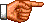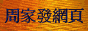# 點算的奧秘：點算問題的遞歸關係

「遞歸關係」(Recurrence Relation，亦譯作「遞推關係」或「遞迴關係」)是除了「容斥原理」、「母函數」和「伯恩賽德-波利亞定理」以外，另一求解「點算組合學」問題的重要工具。在數學上，「遞歸關係」可用來定義某些函數，最常見的例子就是「階乘」(Factorial)的定義。在《點算的奧秘：排列和組合基本公式》中，筆者把「n階乘」(n!)定義為

n! = n × (n − 1) × (n − 2) × ... 2 × 1

 n! = n × (n − 1)! ，若n > 1 1! = 1

2! = 2 × (2 − 1)! = 2 × 1! = 2

 C(n, r) = C(n − 1, r − 1) + C(n − 1, r) ，若n > 0，r > 0，n ≥ r (1) C(n, r) = 0 ，若n < r C(n, 0) = 1 ，對任何非負整數n而言

 C(n + 1, 1) = C(n, 0) + C(n, 1)，即 n + 1 = C(n, 0) + n C(n, 0) = 1

C(2, 2) = C(1, 1) + C(1, 2) = 1 + 0 = 1

 E(n, r) = C(n + r − 1, r) = C(n + r − 2, r − 1) + C(n + r − 2, r) = E(n, r − 1) + E(n − 1, r)

 E(n, r) = E(n, r − 1) + E(n − 1, r) ，若n > 0，r > 0 (2) E(0, r) = 0 ，對任何正整數r而言 E(n, 0) = 1 ，對任何非負整數n而言

 n! n(n − 1)! P(n, r) = ----------- = ------------------------ (n − r)! (n − r)(n − r − 1)! (n − r + r)(n − 1)! = ----------------------------- (n − r)(n − r − 1)! (n − r)(n − 1)! r(n − 1)! = ------------------------ + ------------------------ (n − r)(n − r − 1)! (n − r)(n − r − 1)! (n − 1)! r(n − 1)! = -------------------- + -------------------- (n − r − 1)! (n − r)! = P(n − 1, r) + rP(n − 1, r − 1)

 P(n, r) = P(n − 1, r) + rP(n − 1, r − 1) ，若n > 0，r > 0，n ≥ r (3) P(n, r) = 0 ，若n < r P(n, 0) = 1 ，對任何非負整數n而言

 U(n, r) = nU(n, r − 1) ，若r > 0 (4) U(n, 0) = 1 ，對任何非負整數n而言

 P1(r, n) = P1(r − 1, n − 1) + nP1(r − 1, n) ，若r > 0，n > 0，r ≥ n (5) P1(r, n) = 0 ，若r < n P1(0, 0) = 1 P1(r, 0) = 0 ，對任何正整數r而言

 p(r, n) = p(r − 1, n − 1) + p(r − n, n) ，若r > 0，n > 0，r ≥ n (6) p(r, n) = 0 ，若r < n p(0, 0) = 1 p(r, 0) = 0 ，對任何正整數r而言

 an = 3an−1 + 3an−2 ，若n > 0 a0 = 1，a1 = 4

 a2 = 3a1 + 3a0 = 3 × 4 + 3 × 1 = 15 a3 = 3a2 + 3a1 = 3 × 15 + 3 × 4 = 57 a4 = 3a3 + 3a2 = 3 × 57 + 3 × 15 = 216 □

an = 3an−1 + an−1 − an−2 = 4an−1 − an−2

 an = 4an−1 − an−2 ，若n > 0 a0 = 1，a1 = 4

 a2 = 4a1 − a0 = 4 × 4 − 1 = 15 a3 = 4a2 − a1 = 4 × 15 − 4 = 56 a4 = 4a3 − a2 = 4 × 56 − 15 = 209 □

 an = 2an−1 + n − 1 ，若n > 1 a1 = 0

 a2 = 2a1 + 2 − 1 = 2 × 0 + 1 = 1 a3 = 2a2 + 3 − 1 = 2 × 1 + 2 = 4 a4 = 2a3 + 4 − 1 = 2 × 4 + 3 = 11 □

 an = 2an−1 + 3n−1 − 2n−1 ，若n > 1 a1 = 0

 a2 = 2a1 + 31 − 21= 2 × 0 + 3 − 2 = 1 a3 = 2a2 + 32 − 22= 2 × 1 + 9 − 4 = 7 a4 = 2a3 + 33 − 23= 2 × 7 + 27 − 8 = 33 □